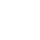# Analog Computer Trainer

Analog Computer Trainer .
he Analog Computer Trainer is a totally solid state electronic device designed to teach the basics of analog computation and to solved various differential equations to engineering & science students. Using this trainer board, students can study the various building blocks used in the analog computation like summing amplifiers, integrating amplifiers, inverting amplifiers & coefficient multipliers. Using this trainer board the students can solve linear equations, simultaneous equations & differential equations with constant coefficients.

Objects:
01. To study building blocks.
1.1. To study summing amplifier (Adder)
1.2. To study the inverting amplifier
1.3. To study difference amplifier (Sub tractor)
1.4. To study summing & difference amplifier (Adder & Sub tractor)
1.5. To study integrating amplifier.
1.6. To study summing integrating amplifier

02. To study analog computer
2.1. To solve linear equations
2.2. To solve simultaneous linear equations
2.3. To solve linear differential equations
2.4. To solve simultaneous differential equations with constant coefficient.
Specifications:
01. No. of summing amplifier : 5
02. No. of Inverting amplifier : 50
3. No. of coefficient multipliers : 10
04. No. of Integrating amplifier : 5
05. Output voltage swing : ±12V
06. Input impedance of each amplifier : 1M
07. Power supply : 3 nos.09. Modes of operation : HOLD, COMPUTE, RESET, GRAPH PLOT & REPETITIVE
Features:
01. FET input Op-Amp. are used.
02. In built power supply
03. Detachable patch board is provided therefore computer may be programmed to solve many kinds of problems.
04. Variable reference voltage controlled by helical potentiometer.
05. Digital voltmeter is provided to set coefficient multiplier and to measure output voltage.
06. Time scaling switch to vary the time constant of integrator
07. Mode selection switch to select mode of operation08. Facility to set initial condition to any desire value.
* Weight : 9 Kg. (Approx.)
* Dimension : W 445 x H 260 x D 470
Other Apparatus Required:
* Cathode Ray Oscilloscope (necessary only in REPETITIVE mode)

### Related Training Products from Indian Manufacturers Suppliers Exporters ...

Tender Related Teaching Lab Products, Scientific Instruments and Educational Equipments like Analog Computer Trainer buy and order from Manufacturers in India